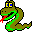#Boost C++ Libraries

...one of the most highly regarded and expertly designed C++ library projects in the world.#connected_components

```// named parameter version
template <class VertexListGraph, class ComponentMap, class P, class T, class R>
typename property_traits<ComponentMap>::value_type
connected_components(VertexListGraph& G, ComponentMap comp,
const bgl_named_params<P, T, R>& params = all defaults);

// there is not a non-named parameter version of this function
```

The connected_components() functions compute the connected components of an undirected graph using a DFS-based approach. A connected component of an undirected graph is a set of vertices that are all reachable from each other. If the connected components need to be maintained while a graph is growing the disjoint-set based approach of function incremental_components() is faster. For ``static'' graphs this DFS-based approach is faster .

The output of the algorithm is recorded in the component property map comp, which will contain numbers giving the component number assigned to each vertex. The total number of components is the return value of the function.

### Parameters

IN: const Graph& g
An undirected graph. The graph type must be a model of Vertex List Graph and Incidence Graph.
Python: The parameter is named graph.
OUT: ComponentMap c
The algorithm computes how many connected components are in the graph, and assigning each component an integer label. The algorithm then records which component each vertex in the graph belongs to by recording the component number in the component property map. The ComponentMap type must be a model of Writable Property Map. The value type shouch be an integer type, preferably the same as the vertices_size_type of the graph. The key type must be the graph's vertex descriptor type.
Python: Must be an vertex_int_map for the graph.
Python default: graph.get_vertex_int_map("component")

### Named Parameters

UTIL: color_map(ColorMap color)
This is used by the algorithm to keep track of its progress through the graph. The type ColorMap must be a model of Read/Write Property Map and its key type must be the graph's vertex descriptor type and the value type of the color map must model ColorValue.
Default: an iterator_property_map created from a std::vector of default_color_type of size num_vertices(g) and using the i_map for the index map.
Python: The color map must be a vertex_color_map for the graph.
IN: vertex_index_map(VertexIndexMap i_map)
This maps each vertex to an integer in the range [0, num_vertices(g)). This parameter is only necessary when the default color property map is used. The type VertexIndexMap must be a model of Readable Property Map. The value type of the map must be an integer type. The vertex descriptor type of the graph needs to be usable as the key type of the map.
Default: get(vertex_index, g). Note: if you use this default, make sure your graph has an internal vertex_index property. For example, adjacenty_list with VertexList=listS does not have an internal vertex_index property.
Python: Unsupported parameter.

### Complexity

The time complexity for the connected components algorithm is also O(V + E).